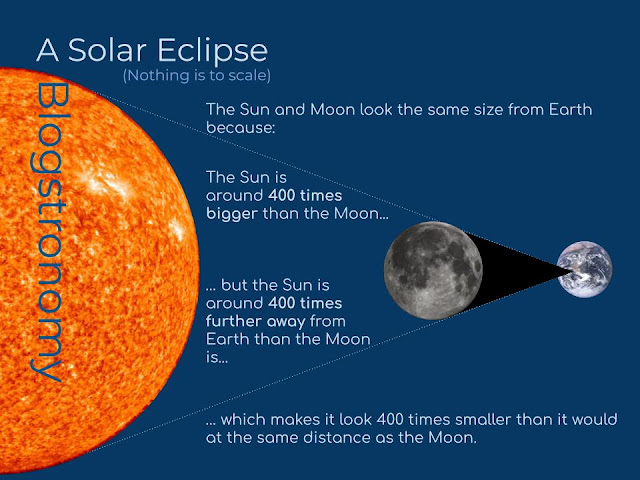### What Does a Solar Eclipse Look Like From the Moon?

Question posed by a couple of Mice. This question involves the Sun, so my usual warning applies: do not ever, under any circumstances, look directly at the Sun.

This is an interesting question, not least because there's more than one way to interpret it...

## What is a Solar Eclipse?

A solar eclipse is an astronomical event which happens (literally) periodically when the Earth, Moon and Sun line up in such a way that the Moon completely covers the Sun from our point of view, with the fact that the Moon and Sun appear almost exactly the same size being part of what what makes them so impressive. Here's a reminder of why:So one way of interpreting this question is "what would we see from the Moon when the Earth is experiencing a solar eclipse?".

However, if we're on the Moon, wouldn't we be more interested in the situation when it is the Earth getting in the way of the Sun from our point of view standing on the Moon? I think this is probably what the Mice were thinking of when they asked their question:

## "What would we see from the Moon if the Earth was eclipsing the Sun?"

It might help to think about how this situation would differ from the solar eclipses that we're used to, so the image a bit further down the page compares the two. The first part of the diagram shows the Moon eclipsing the Sun from the point of view of someone standing in the right place on Earth. I can't remember how far the Moon is from the Earth, and to answer this question it doesn't matter, so I've been lazy and just called it "$x$". I do know that the Sun is about 400 times further away, though: that's the important thing.

I also know that the diameter of the Sun is about 400 times the diameter of the Moon: that's easy to remember because the numbers are the same, which is a really cool and also strange coincidence - but very definitely a coincidence.

So to work out how big the Sun looks compared to the Moon in a normal eclipse situation we can do this calculation:

$Scale = \frac{relative.size}{relative.distance}$

Where "$relative.size$" is how many times bigger it is and $relative.distance$ is how many times further away it is. This works because if you move something twice as far away from you it looks half as big; if you move it 3 times further away it looks one third of the size; and so on.

In the case of a solar eclipse as viewed from Earth, we can substitute the following values:

$Scale = \frac{400}{400} = 1$

So it looks 1 times the size, which means it looks the same size: that's exactly what we expect from a total eclipse of the heart Sun."But we know all about eclipses viewed from Earth!",

I hear you shout;

"Give us the Moon goods!"

We can use the same calculation to work out how big the Earth would look if it is the thing blocking the Sun from our vantage point on the Moon. We just have to work out which numbers to use...

$Scale = \frac{relative_size}{relative_distance}$

The second situation in the diagram above is the one we're really interested. If the Earth is now blocking the Sun's light from reaching our place on the Moon, then the Moon has moved 180° in its orbit and is now on the other side of the Earth. The Earth is the same distance from the Sun as it was before (which we called $400 \times x$, where "$x$" was the distance between the Earth and Moon that we couldn't remember. The Moon is now that much further away from the Sun, so the Sun is 401 times further from the Moon than it is from the Earth. That's not a big difference, so shall we keep the numbers simple and estimate it as 400? OK then.

The other thing we need to know is how many times bigger the Sun is than the Earth, this time. It turns out that the Sun's diameter is only about 100 times that of the Earth, so our new calculation is:

$Scale = \frac{400}{100} = 4$

That means that from the Moon the Earth looks about 4 times the size of the Earth. Here's a comparison:If you're interested in that other question...

## "What would we see from the Moon when the Earth is experiencing a solar eclipse?"

... there are photos! The one below was taken by a Chinese microsatellite called Longjiang 2 on 2nd July 2019 during a total eclipse visible from South America. You can see that it looks very much like our second image above, except with a fuzzy edge.A solar eclipse image taken from lunar orbit by DSLWP-B. Credit: @BY2HIT on Twitter